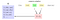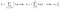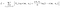TL;DR

In this 3-part blog series we present a unifying perspective on pre-trained word embeddings under a general framework of matrix factorization. The most popular word embedding model, Word2vec, has traditionally been presented as a (shallow) neural network. By the end of this blog post series, you will have learned how to compute the same Word2vec embeddings with a single matrix factorization. In the following parts, we’ll show you how to simply compute that matrix factorization using a standard deep learning library such as TensorFlow.

This series is based on my recent work pursued at Mila (Quebec AI Institute) in collaboration with Edward Newell (PhD), currently under review. Note that the proof provided in this post was discovered by Edward, and the originality of the idea must be credited to him. It proceeds as follows:

Part 1: Introduction and motivation; check it out!

Part 2: In this post, we will describe the full correct matrix factorization generalization for Word2vec, providing the exact form of the algorithm.

Part 3: In the last part of this series, we will present practical experimental results verifying the correctness of our explicit matrix factorization formulation of Word2vec, providing example code on how simply implementable matrix factorization is by using existing deep learning libraries. Check it out!

Introducing MF-SGNS

Word2vec’s most commonly used variant is Skip-Gram with Negative Sampling (SGNS), which we discussed last time. SGNS is a sampling-based algorithm with a running-time dependent on the size of the corpus — it has to scan the entire input corpus at every epoch. Its counterpart is the matrix-factorization formulation we will derive in this post, MF-SGNS, which has a time complexity independent of the corpus size, but dependent on the vocabulary size (naively implemented, a quadratic dependency).

To derive MF-SGNS, we need to reason about the following questions:

• What is SGNS really doing at every step along the corpus?
• How can we accumulate (or, count) the repeated updates?

This is best understood through an example. Let’s recall that SGNS defines a context window of size w, and that this context window determines what pairs of words we will be updating upon.Example of how we accumulate all the term-context pairs by scanning over the corpus of text.

In the image above we provide an example of how this scanning over the corpus occurs with a context window size of w=1. As we read over the corpus, we store every pair that we observe into a (very, very, very, long) list called Ω (mega). The items in Ω are the positive samples, and it will be filled with approximately |Ω| = 2 * w * len(corpus) samples; recall that the corpus length is often on the order of billions of words!

Note that the definition of the context window is symmetric, and that SGNS treats words separately as terms and contexts. In this example, “is” is the current term t, and “the” is the current context c, which will be filled into the 5th slot of Ω. Recall that SGNS will learn two sets of embeddings, one for the terms and one for the contexts, and (normally) SGNS will only output the term vectors, which are what we call the “word embeddings”.

Positive Samples + Negative Samples

It is now worth re-examining the loss function of SGNS as presented last time in a new light, based on every term-context pair in Omega. Let w_t refer to the term vector for the word corresponding to the term t, and let c_c refer to the context vector for the word corresponding to the context c. We then have the following as the global (i.e., across the entire corpus) loss function for SGNS:Loss function for SGNS over the entire corpus. σ is the logistic sigmoid function.

The inner summation represents the negative sampling of SGNS, where, for every positive sample in Ω, k negative samples are drawn from the context unigram distribution U of the corpus. This distribution is easy to define by counting the unigram statistics from the corpus, although Mikolov et al.  found that it was necessary to use exponential smoothing on this distribution in order to obtain good results (see Levy & Goldberg (2015) for details ).

This means that the word embedding w_t will receive gradient updates positively from c_c and negatively for all k negative samples c_n. Additionally, each context vector will receive one gradient update from w_t; if this is confusing, consider the partial derivatives of L with respect to each of the vectors w_t, c_c and c_n. This is why increasing the number of negative samples k with SGNS results in faster learning, since w_t will receive exactly k+1 updates from the gradient at every step of learning.

To turn SGNS into matrix factorization, we must ask, for every term-context pair (i,j):

• How many times was (i,j) positively sampled? And,
• How many times was (i,j) negatively sampled?

Answering these questions requires us to reason about what it means to “count” things.

Counting up the Corpus Statistics

The first half of counting will be easy! We can write a very trivial Python code to represent how we will count from Omega (using the very helpful collections.defaultdict object). Namely, we will be counting how many times (i,j) occurs in omega by hashing every (i,j) pair to its count; we will call the number of positive (i,j) samples N_ij:

Nij_counts = defaultdict(int)
for (i,j) in Omega:
Nij_counts[ (i,j) ] += 1

And that’s it! We’ve now counted all of the positive term-context samples.

Let N be the total length of Omega; then, the probability of (i,j)-cooccurrence is just: P(i,j) = N_ij / N. This will be very important later.

Counting up the negative samples is a bit trickier. We have to now reason about what the context unigram distribution U looks like. This can be done by marginalizing our Nij_counts above. Marginalizing allows us to determine: how many times do we expect to see a context j? We call this value N_j, the context counts, and we will also need to count the terms to get the term counts N_i. (Of course, this can all be implemented in a much more efficient way than I am presenting below.)

Ni_counts = defaultdict(int)
Nj_counts = defaultdict(int)
for (i,j), N_ij in Nij_counts.items():
Ni_counts[ i ] += N_ij
Nj_counts[ j ] += N_ij

Note, most of the time we use a symmetric context window, so N_i and N_j will be equivalent for all i=j, and N_ij will be a symmetric (& very sparse) matrix. However, as noted above, the context unigram distribution will be smoothed by SGNS, so it is helpful to define it separately. Additionally, there may be cases where it would make sense to define an asymmetric context window; e.g., perhaps in a text recommendation system we would only want our term-vectors to model contexts that come after the current word.

Now, we have the context unigram distribution! We can turn this into a probability distribution by normalizing it over the length of Omega; that is, we can get the probability of a context j by simply defining P(j) = N_j / N, for every N_j item. Similarly, we can get the probability of seeing a term i by defining P(i) = N_i / N.

How many negative samples?

Given that we now know the distribution from which negative samples are sampled (that is, we know the U in the inner summation in the loss function above) we can answer the fundamental question: how many times do we expect to draw a certain term-context pair (i,j) as a negative sample?

Answering this requires answering two sub-questions. (1) How many times will we see term i while iterating over the loss function above? (2) How many times will we see context j, given that we saw that term i?

Well, we’ve already counted these things! (1) We know we will see term i exactly N_i times. (2) We know that sampling from the unigram distribution is independent of the current term, thus, we can expect to negatively sample a context j according to its probability in U, that is, according to the probability N_j / N. But, we will draw k negative samples at every step, so we multiply this probability by k.

Therefore, we will see (i,j) drawn as a negative sample exactly N_i * k * N_j / N times.

We’ve now answered our questions above:

• (i,j) will be drawn as a positive sample exactly N_ij times;
• (i,j) will be drawn as a negative sample exactly k * N_i * N_j / N times.

We now have everything we need to turn SGNS into matrix factorization.

The Matrix Factorization formulation of SGNS

If we accumulate all repeated terms in Omega, we realize that we are going to do exactly N_ij positive sampling updates for an (i,j) pair; similarly, for this (i,j) pair, we will be doing exactly k * N_i * N_j / N negative sampling updates. Therefore, we can rewrite the loss function as one that iterates over all (i,j) pairs in the vocabulary set squared V x V:The loss function of SGNS, written in the form required for matrix factorization; i.e., MF-SGNS.

This non-convex loss function can be very easily implemented in any automatic differentiation toolkit (such as TensorFlow) in order to take advantage of state-of-the-art solvers such as Adam — — we will go over an exact implementation of this in the next post of this 3-part series.

For now, it is worth noting that matrix factorization will require all w_i * c_j dot products to be computed in order to properly use this loss function. This can be very easily implemented with a large matrix multiplication. If W is a V x d matrix of all the term vectors w_i, and C is a d x V matrix of all the context vectors c_j, then all we will need to do is compute W @ C to get the V x V matrix of dot products for this loss function.

While this loss function presents the exact form needed for a matrix factorization implementation of SGNS, we will actually need to dig deeper in order to determine the obvious question: what matrix is being factorized?

What matrix is being factorized?

With the help of some clever algebra and the laws of calculus, we can differentiate L with respect to the dot product itself to determine the form of the gradient descent updates when using this loss. From there, we can think about what would be necessary for the derivative to be zero — for the model to reach a local optimum for one (i,j) pair. We will spare the details of this derivation for now; the partial derivative (for a specific (i,j) pair) is:Partial derivative of MF-SGNS loss function, with respect to the dot product.

At first glance, this does not look like it is particularly intuitive or revealing. Nonetheless, let’s first note the two components of this partial derivative: on the left-hand side there is simply a multiplier, a scaling on the update; on the right hand side we have a measure of difference between the model’s dot product and the logarithm in the sigmoid. One can easily verify that if the model’s (i,j) dot product is equal to log (N N_ij / k N_i N_j), the derivative will be zero, and the model will have reached a local optimum (more precisely, it will have reached a stationary point of the loss function).

Extending this reasoning to the entire loss function over all (i,j) terms, if every dot product equals the corresponding (i,j) logarithm value, then we will have solved the optimization. Therefore, the matrix being factorized must be filled with these log (N N_ij / k N_i N_j) terms in every cell.

But what is this log (N N_ij / k N_i N_j) term?

Let’s recall the definition of pointwise mutual information, PMI:

Using our corpus statistics that have already been computed above, we have already defined each of these probabilities:

So, plugging them back in the equation for PMI we get:

But this is almost exactly our term above, except we have an extra k in the denominator! Therefore, all we have to do is apply the log rule, and we finally obtain:

That is, we have found that SGNS factorizes the matrix of shifted-pointwise mutual information statistics, PMI — log k; exactly the same finding as Levy and Goldberg (2014) !

Conclusion

Not only have we found out that SGNS factorizing this PMI matrix — like Levy and Goldberg — but we also have derived the loss function that allows us to factorize this matrix.

Fundamentally, this loss function uses the logistic sigmoid, which naturally attenuates to deal with the problem of when PMI = -∞; if that happens, there’s no divergence with this loss function, we will just be computing σ(-∞) instead, which is just 0!

Recall from last time that Levy and Goldberg’s original finding had two drawbacks: (1) they did not use the correct loss function (they used SVD instead); and, (2) they did not factorize the correct matrix, by virtue of using the wrong loss function (they used positive-PMI instead, which gets rid of about half of the data and all the negative infinities).

Our solution solves both of these problems: (1) we have found the correct loss function, which can be used with any automatic differentiation software; and, (2) we factorize the true shifted-PMI matrix, which is not problematic since the loss function naturally deals with the negative infinities in PMI.

Next time, we will examine how to implement this in a deep learning toolkit, we will look at empirical results comparisons between SGNS and our proposed MF-SGNS (spoiler alert, they perform equivalently, and sometimes MF-SGNS is even better), and we will finally provide a commentary on the pros and cons of using matrix factorization, concluding this 3-part series.

[1.] Mikolov, Tomas, et al. “Distributed representations of words and phrases and their compositionality.” NeurIPS. 2013.

[2.] Levy, Omer, Yoav Goldberg, and Ido Dagan. “Improving distributional similarity with lessons learned from word embeddings.” TAACL. 2015.

[3.] Levy, Omer, and Yoav Goldberg. “Neural word embedding as implicit matrix factorization.” NeurIPS. 2014.

Written by

Kian Kenyon-Dean

Our adventures in improving the world with deep learning

Welcome to a place where words matter. On Medium, smart voices and original ideas take center stage - with no ads in sight. Watch
Follow all the topics you care about, and we’ll deliver the best stories for you to your homepage and inbox. Explore
Get unlimited access to the best stories on Medium — and support writers while you’re at it. Just \$5/month. Upgrade